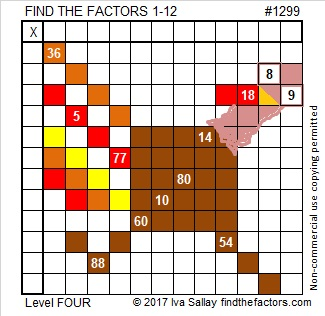# 1299 Is This Puzzle a Real Turkey?

Happy Thanksgiving, everyone!

Turkeys run but they cannot hide. They all will eventually end up on somebodies’ table. There doesn’t seem to be much of a mystery about that, but I’ve created a mystery level puzzle for today anyway. I promise it can be solved using logic and the basic facts in a 12 × 12 multiplication table.Now I’ll share some facts about the number 1299:

• 1299 is a composite number.
• Prime factorization: 1299 = 3 × 433
• The exponents in the prime factorization are 1 and 1. Adding one to each and multiplying we get (1 + 1)(1 + 1) = 2 × 2 = 4. Therefore 1299 has exactly 4 factors.
• Factors of 1299: 1, 3, 433, 1299
• Factor pairs: 1299 = 1 × 1299 or 3 × 433
• 1299 has no square factors that allow its square root to be simplified. √1299 ≈ 36.041641299 is the hypotenuse of a Pythagorean triple:
435-1224-1299 which is 3 times (145-408-433)

Stetson.edu informs us that 8¹²⁹⁹ ≈ 1299 × 10¹¹⁷⁰. You can see it for yourself on a computer calculator!